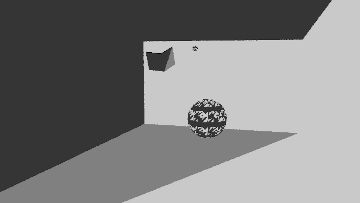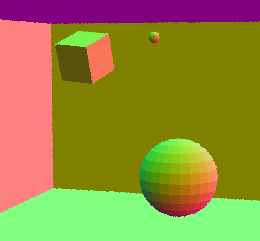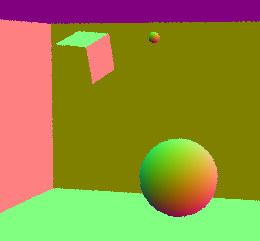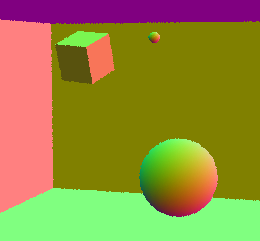# Solved Getting poly normal and CreatePhongNormals()

Hi folks,

I'm trying to calculate a polygon's normal. I've read CreatePhongNormals() can return an array of (vertices point?) normals if there's a Phong tag. How do I use that to calculate a normal at poly UV?

``````// psuedo code
po = (PolygonObject*)obj;
SVector *norms = po->CreatePhongNormals();

if(norms)
{
int ply = 4;  // let's get normal for polygon 4(5), points abc
Vector uv;  // the uv spot of the normal to get, on ply

Vector normal = (norms->operator[](ply*4) + norms->operator[](ply*4+1) + norms->operator[](ply*4+2)) / 3;

// do something with normal, etc.

GeFree(norms);
}
``````

Would like to include blending across the polygon if possible (i.e. the normal at spot uv). Not worried about speed, just learning.

WP.

Hi,
CreatePhongNormals only create the vertex normals accordingly to the phong tag.
This tag, display those normals, you can change the phong tag setting and a plane's shape to understand them a bit better.

``````import c4d
#Welcome to the world of Python
import random

colors = []

def draw (bd):
global colors

obj = op.GetObject()
if obj is None:
raise ValueError("can't retrieve the object")
# retrieves the information about the object
points = obj.GetAllPoints()
normals = obj.CreatePhongNormals()
# scale the normals
normals = [i * 100 for i in normals]

polyCnt = obj.GetPolygonCount()

for i in range(polyCnt):
# gets the polygon cpoly structure
cpoly = obj.GetPolygon(i)

bd.SetPen(colors[i])

pointsIndexes =  [cpoly.a,  cpoly.b,  cpoly.c,  cpoly.d]
for j,  pointIndex in enumerate(pointsIndexes):
# retrieves the point position
vecStart = points[pointIndex]
# retrieves the normal direction. (add the point position)
vecEnd = vecStart + normals[i * 4  + j]
bd.DrawLine (vecStart, vecEnd, c4d.NOCLIP_D )

def main():
# generates enough random color (1 per poly)
global colors
random.seed(183446)
obj = op.GetObject()
if obj is None:
raise ValueError("can't retrieve the object")
polyCnt = obj.GetPolygonCount()
for i in range(polyCnt):
colors.append(c4d.Vector (random.random(), random.random(), random.random()))
``````

Those phong normals are an average of every vertex normals a vertex have. (a vertex have as many normal than the number of polygon it's part of)
According to the angle you set in the phong tag, the vertex will have 1 or several normals.

doing it yourself :

• you have to calculate the normal of all your polygons.
• calculate the average of each vertex normal.
• for each uv's coordinate you want, calculate the barycentric coordinate. That will give you the ratio for each point.
• calculate the bilinear interpolation in 3D.

Cheers,
Manuel

MAXON SDK Specialist

MAXON Registered Developer

Just FYI: To calculate the normal of a single `CPolygon`, there is CalcFaceNormal().

What version of Cinema 4D are you using? `GeFree()` is pretty outdated.

i keep forgetting this CalcFaceNormal...

MAXON SDK Specialist

MAXON Registered Developer

Thanks Manuel and PStudent,

I'm going around in circles. When I try blending between the 3 Phong normals, it comes out in variants like this:The following is the code I use to calc the Phong normal:

``````//... hit test function here...
// f = 1 / area
Vector uvw;
uvw.x = f * s.Dot(h);
uvw.y = f * dir.Dot(q);
uvw.z = 1 - uvw.x - uvw.y;
//... end of hit test, return true if hit, uvw passed over...

// if hit function returns true, use uvw to calc normal at hit spot
// norms = CreatePhongNormals()
// let's test first polygon in poly 4(5)
Vector normal = uvw.x * norms->operator[](4*4) + uvw.y * norms->operator[](4*4+1) + uvw.z * norms->operator[](4*4+2);
// repeat for second tri in quad, if exists
// etc

``````

I can get the plain polygon face normal fine. But blended ones I can't get my head around. Where am I going wrong here?

WP.

Hi,

at least to me it is not quite clear what you are trying to do. One reason is that there are quite a few symbols floating around in your code which are not self-explanatory (what are `s, q, h` and `dir`?). I also do not quite understand why you are computing something into the vector `uvw` what seems to be a linear combination.

If you are interested just in the polygonal normal, you can compute that by evaluating the cross product of the vertices of a corner of your choice in the polygon in a clockwise fashion (e.g. `d-a % b-a` for the vertex `a` in a four point polygon). In non-planar polygons you will have to evaluate two vertices, or more realistically all four, since that is faster than finding "the right ones", and compute the arithmetic mean of them.

If you want to to interpolate between the already interpolated point normals (a.k.a. Phong normals), a simple bi-linear interpolation between these four normals should be enough. Where the `u` and the `v` coordinates of your texture data (or wherever they are coming from) are the parameters for the respective interpolation on that axis. You won't need barycentric coordinates or something like that.

Cheers,
zipit

MAXON SDK Specialist
developers.maxon.net

same here, not sure what you are trying to achieve at the end.

MAXON SDK Specialist

MAXON Registered Developer

Thanks Zipit / Manuel,

@zipit said in Getting poly normal and CreatePhongNormals():

If you want to interpolate between the already interpolated point normals (a.k.a. Phong normals), a simple bi-linear interpolation between these four normals should be enough

Yep - that's what I'm trying to do!!But I have a way with code that makes simple things really difficult. Whatever I try isn't working. I got to this:but can't figure out the bi-linear interpolation. This is where I'm going around in circles. Maybe we take a step back for a moment and do this in two steps.

Step 1: I have a function that tests each polygon triangle using the Moller-Trumbore algorithm. Assuming the hit is true, how do I calculate the UV? I'm asking this step to check if I'm setting UV right (which, is where some of the code above comes from).

Step 2: how do I use UV to interpolate between Phong normals a,b and c (triangle)?

The end goal is to draw a normals map.

WP.

Hi,

a bilinear interpolation is quite straight forward. If you have the quadrilateral Q with the the points,

``````c---d
|   |
a---b
``````

then the bilinear interpolation is just,

``````ab = lerp(a, b, t0)
cd = lerp(c, d, t0)
res = lerp(ab, cd, t1)
``````

where `t0, t1` are the interpolation offset(s), i.e. the texture coordinates in your case (the ordering/orientation of the quad is obviously not set in stone). I am not quite sure what you do when rendering normals, but when you render a color gradient, in a value noise for example, you actually want to avoid linear interpolation, because it will give you these ugly star-patterns. So you might need something like a bi-quadratic, bi-cubic or bi-cosine interpolation, i.e. pre-interpolate your interpolation offsets.

If I am not overlooking something, this should also work for triangles when you treat them as quasi-quadrilaterals like Cinema does in its polygon type.

Cheers,
zipit

MAXON SDK Specialist
developers.maxon.net

Hi,

Moller-Trumbore

what I forgot to mention, but I already hinted at in my previous posting, is that you did not state in which space your interpolation coordinates are formulated. When you talk about uv-coordinates I am (and probably also everyone else is) assuming that you have cartesian coordinates, just like Cinemas texture coordinate system is formulated in.

If you have another coordinate system, some kind of linear/affine combination like your code snippet suggests, then the interpolated normal is just the linear combination of the neighbouring normals (I think that was what you were trying to do in that code).

If this fails, you should either check your Möller-Trumbore code for errors (Scratch-Pixel has a nice article on it and how to calculate correct affine space/barycentric coordinates for it), or more pragmatically use Cinema's `GeRayCollider` instead, which will also give you texture coordinates.

I am not so well versed in the C++ SDK, maybe there is even a more low level (i.e. triangle level) version of `GeRayCollider` there, i.e. something where you do not have the overhead of casting against a whole mesh.

Cheers,
zipit

MAXON SDK Specialist
developers.maxon.net

Oooh..... I feel like such a dope. I had almost everything right to begin with, except I was accessing the returned CreatePhongNormals() SVector incorrectly. I was using operator()[] when I should have been using ToRV(). Here's a smoothed result:Thanks guys, we got thereOne last thing, how do I 'globalise' the normals? They look local to me (see floating cube, it's slightly rotated but has the same shading as walls in the background)?

WP.

Hi,

all polygonal data is in object space. So if you want your normals to be in global/world space, you will have to multiply them with the frame of the object they are attached to. To get the frame, you will only have to zero out the offset of the global matrix of the object and then normalise the axis components.

Cheers,
zipit

MAXON SDK Specialist
developers.maxon.net

Thanks zipit,

makes sense being local. Zeroing out and multiplying by the frame matrix does it:Thanks for your time contributors, been a big help.

WP.

hi

can we considered this thread as resolved ?

Cheers,
Manuel

MAXON SDK Specialist

MAXON Registered Developer

Thanks Manuel,

I didn't realise I had to mark the thread as solved. Done.

WP.

@WickedP Uhh, Ohh what are you coding there is that a brdf map shader ? I am searching for something like this !

https://alastaira.wordpress.com/2013/11/26/lighting-models-and-brdf-maps/

Hi @mogh,

interessting, but not what i hoped for ...